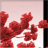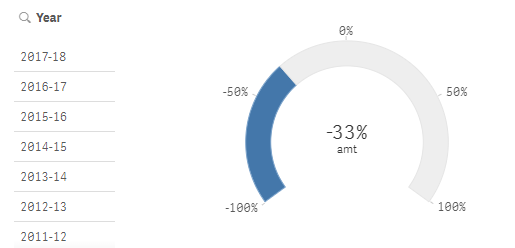# App Development

Announcements
cancel
Showing results for
Did you mean:Partner

## Dynamic selection

Hello Community,

I want to get sum of amount for current selection of year and then subtract value of its previous year from that.

Below expression works in static manner, i want it to work dynamically based on selection of years.

((((sum({<Reference={'1','2','3'},[FY Year Name]={'2017-18'} >}Amount))-

(sum({<Reference={'1','2','3'} ,[FY Year Name]={'2016-17'}>}Amount)))/(sum({<Reference={'1','2','3'} ,

[FY Year Name]={'2016-17'}>}Amount))))

This is the result of the above expression, but i want it to change based on selection in year filter.1 Solution

Accepted SolutionsPartner

Your year field is a text one, so it would be better if you transform it into a number format for better handling.

Instead of putting a year comparison in the year field (2017-18) just do a normal number, like 2017 and 2018 as separate rows in the field and the set expression would be like:

((((sum({<Reference={'1','2','3'},[FY Year Name]={'\$(=Max(Year))'} >}Amount))-

(sum({<Reference={'1','2','3'} ,[FY Year Name]={'\$(=Max(Year)-1)'}>}Amount)))/(sum({<Reference={'1','2','3'} ,

[FY Year Name]={'\$(=Max(Year)-1)'}>}Amount))))

3 RepliesPartner

Your year field is a text one, so it would be better if you transform it into a number format for better handling.

Instead of putting a year comparison in the year field (2017-18) just do a normal number, like 2017 and 2018 as separate rows in the field and the set expression would be like:

((((sum({<Reference={'1','2','3'},[FY Year Name]={'\$(=Max(Year))'} >}Amount))-

(sum({<Reference={'1','2','3'} ,[FY Year Name]={'\$(=Max(Year)-1)'}>}Amount)))/(sum({<Reference={'1','2','3'} ,

[FY Year Name]={'\$(=Max(Year)-1)'}>}Amount))))Partner
Author

Thank You Felip , it works perfectlyPartner

Glad it helped.Tags